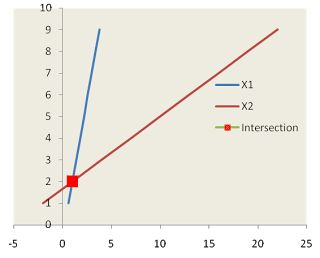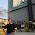# Excel : Intersection of two linear straight lines

Most of us must find intersection of two linear straight lines with pen and paper during school days. In this article, we will see how to solve it with Excel.

To find intersection of two straight lines:
• First we need the equations of the two lines.
• Then, since at the point of intersection, the two equations will have the same values of x and y, we set the two equations equal to each other. This gives an equation that we can solve for x
• We substitute that x value in one of the line equations and solve it for y
Let the lines follow a system of linear equations :

y1=m1x1+c1 and y2=m2x2+c2
Where
m1: Gradient or Slope of line1,
c1: Intercept of line1,
m2: Gradient or Slope of line2,
c2: Intercept of line2

Let the point of intersection be s and t. They must satisfy the above 2 equations.

t=m1s+c1 and t=m2s+c2 . Then m1s+c1=m2s+c2
s= (c2-c1)/(m1-m2) , t=m1*s+c1
Use SLOPE and INTERCEPT functions to replicate these equations in excel.
s=(INTERCEPT(y-values2,x-values2)-INTERCEPT(y-values1,x-values1))/(SLOPE(y-values1,x-values1)-SLOPE(y-values2,x-values2))
t =SLOPE(y-values1,x-values1)*X+INTERCEPT(y-values1,x-values1)

For example : I take the following equations :

y1 = 2.5x1 - 0.5
y2=-0.5x1+1.67

Based on the above equations i created data for y1 and y2 using same values on X1.

STEP I:

To find the intersection point :

Apply the following formulae :

X=(INTERCEPT(y-values2,x-values2)-INTERCEPT(y-values1,x-values1))/(SLOPE(y-values1,x-values1)-SLOPE(y-values2,x-values2))

and Y =SLOPE(y-values1,x-values1)*X+INTERCEPT(y-values1,x-values1)

STEP II:
Insert Scatter with Straight lines chart and mark intersection point ( Right click on lines >> Format Data series >> Marker Options / Fill )Excel : Intersection of 2 linear Straight Lines
Intersection between linesShare
Related PostsDeepanshu founded ListenData with a simple objective - Make analytics easy to understand and follow. He has over 10 years of experience in data science. During his tenure, he has worked with global clients in various domains like Banking, Insurance, Private Equity, Telecom and Human Resource.

7 Responses to "Excel : Intersection of two linear straight lines"
1.Very good tutorial, helped a lot today! Thank you :)

2.hello,

Let say i have a sinusoidal equation, y = A sin wt and a straight line equation, y = mt + c. how do i determine the intersection between those graphs?

3.Sorry, I am slow and got little from it. Perhaps, some practical examples would help. I have home with a roof with a 6:12 slope. I want to add a porch with a 4:12 slope. The porch (with eave) is 11.5 feet wide. How long will the rafter be from the porch's eave up the main roof to the interception point?

4.Thank You.

5.Hi, sir please tell us about yourself. Thanks a lot for this website.

6.Thank you very much

7.MAN I LOVE YOU!
Lifesaver

Next → ← Prev